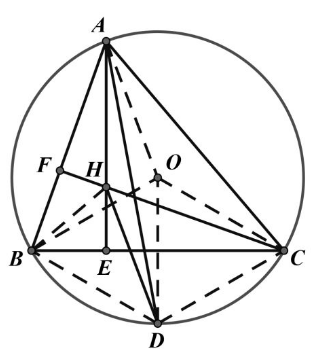【答案】 简证: 易证 $\angle B H C=120^{\circ}, \angle B O C=120^{\circ}, \therefore B 、 H$ 、
O、 $C$ 四点共圆。
$D B=D O=D C, \therefore D H=D O=O A$, 又 $A H / / O D, \therefore$
$\triangle H D O$ 是菱形
$\therefore A H=H D, \triangle A H D$ 为等腰三角形。